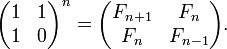# Fibonacci numbers in J

Example for versions j602

Maxtrix closed form

``````load 'printf'

mat =: 1 1,.1 0
fibm=: 3 : '1 { , mat&(+/ . *)^:y mat'"0
fstr=: '...' ,~ ,~^:4 '%d, '
fstr printf fibm >:i.16
``````Fibonacci Matrix Closed Form